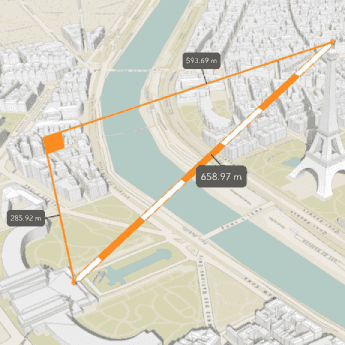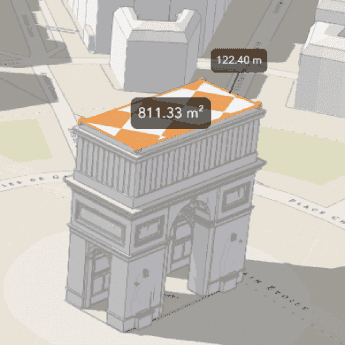# 3D measurement

Measure distance and area interactively in 3D.

## What is a 3D measurement analysis?

A 3D measurement is a type of operation that calculates and displays measurements of elements in a 3D scene. 3D measurement operations can measure the direct, vertical, and horizontal distance between points, as well as the area and perimeter of polygons.

A measurement analysis returns the value of the measurement it performs, as well as a feature representing the distance or area measured. The numerical results of the analysis will be reported in the unit system you specify, and the scale of the unit will automatically match the scale of the measurement.

Real-world use cases for 3D measurement analysis include the following:

• Measuring the actual size of countries and states, which are often distorted by map projections.
• Measuring the topographic prominence of hills and mountain ranges.
• Visualizing the distance between map features to gain a deeper understanding of spatial relationships.
• Determining the size and shape of real estate plots.
• Designing urban structures.

## Types of 3D measurement operations

The following table describes the different types of 3D measurement operations:

OperationDescriptionExample
`LocationDistanceMeasurement`, `DirectLineMeasurementAnalysis`Measures direct, vertical, and horizontal distance between two point locations in a scene.`AreaMeasurementAnalysis`Measures the area and perimeter of a 2D polygon. Only available in ArcGIS Maps SDK for JavaScript.### Distance measurement

A distance measurement analysis is a type of measurement analysis that calculates and displays the distance between a 3D start and end point. The analysis evaluates the vertical, horizontal, and direct distances between the two points and renders a measurement visualization in the scene view.

If the length of the distance line exceeds 100km, the measurement method will be geodesic to account for the Earth's curvature. Otherwise, the analysis will be a planar measurement.

### Area measurement

An area measurement analysis is a type of measurement analysis that calculates the area of a polygon in a scene. The analysis evaluates the surface area and perimeter of a polygon and renders a measurement visualization in the scene view. The measurement polygon can have points at differing heights (`z` values), but must be two-dimensional.

If a side of the shape's perimeter exceeds 100km, the measurement method will be geodesic to account for the Earth's curvature. Otherwise, the analysis will be a planar measurement.

## How to perform a 3D measurement

The following are the basic steps to performing 3D distance and area measurement with ArcGIS APIs.

### Distance measurement

1. Create a scene view and set its extent to the desired location.

2. Identify the segment you want to measure and create two points to mark its beginning and end.

3. Create a `LocationDistanceMeasurement` and set its start and end to the points you created.

4. Add the `LocationDistanceMeasurement` to a new `AnalysisOverlay`.

5. Add the `AnalysisOverlay` to the scene view to display the results.

### Area measurement

1. Create a scene view and set its extent to the desired location.

2. Create a new `AreaMeasurementAnalysis` operation, either directly or through the `AreaMeasurement3D` widget.

3. Supply an area for the operation to measure, either by providing an existing polygon or by sketching a new one through the `AreaMeasurement3D` widget.

4. Add the operation to the scene view to view the measurement results.

## Code examples

### Distance measurement

This example calculates and displays measurements of buildings in New York City. You can create 3D Distance measurements either by directly using the analysis operation or by using the JavaScript `DirectLineMeasurement3D` widget.

ArcGIS Maps SDK for JavaScriptArcGIS Maps SDK for JavaScriptArcGIS Maps SDK for .NETArcGIS Maps SDK for JavaArcGIS Maps SDK for .NET (C#)ArcGIS Maps SDK for Qt (C++)ArcGIS Maps SDK for Qt (QML)
Use dark colors for code blocksCopy

``````              activeWidget = new DirectLineMeasurement3D({
view: view
});
``````

### Area measurement

This example calculates and displays the area of different real estate parcels when they are clicked. It calculates area using the `AreaMeasurementAnalysis` operation, rather than the AreaMeasurement3D widget.

Use dark colors for code blocksCopy

``````        // create a AreaMeasurement object and add it to the `view.analyses`
const areaMeasurementAnalysis = new AreaMeasurementAnalysis();

view.when(() => {
const hitTestLayers = view.map.layers.filter((layer) => layer.title === "Parcels");
view.on("click", async (event) => {
// remove the current measured geometry from the layer when the user clicks on the map
areaMeasurementAnalysis.geometry = null;
// get results from the "Parcels" layer
const hitTestResult = await view.hitTest(event, { include: hitTestLayers });
if (hitTestResult.results.length > 0) {
const geometry = hitTestResult.results.graphic.geometry;

// pass the polygon geometry to the areaMeasurementAnalysis to display a new measurement
areaMeasurementAnalysis.geometry = geometry;

}
});
});
``````